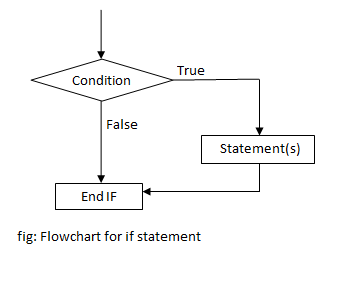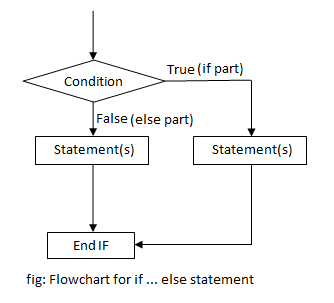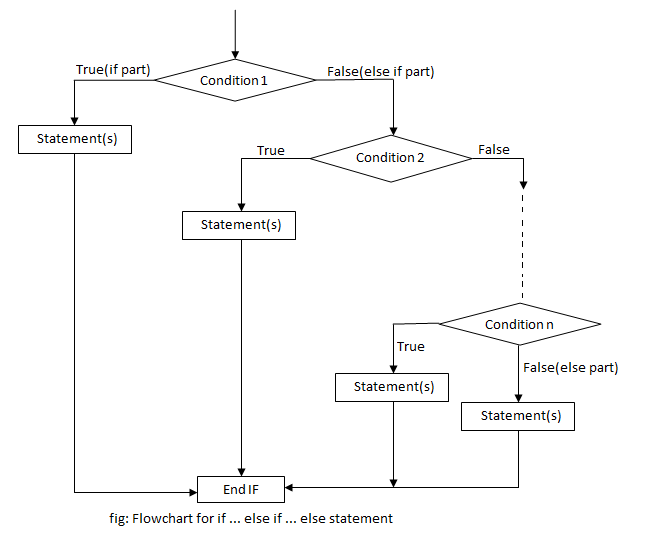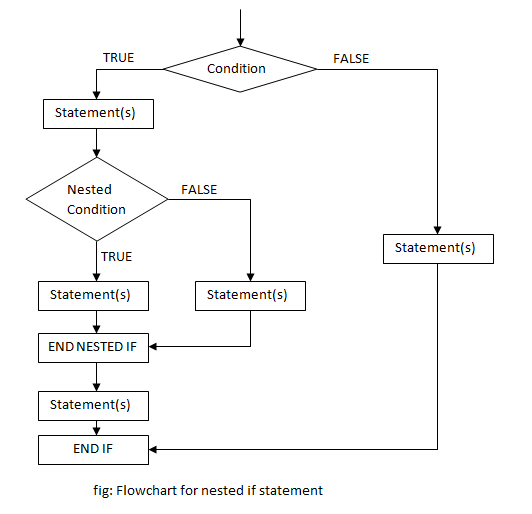# if statement in C Programming

Decision making is an important part of programming. Every programming language supports decision making statements allowing programmers to branch according to the condition. In C programming language, if statement is used to check condition and make decision. The decisions or statements are enclosed inside curly braces, however if only a single statement has to be executed, curly braces are not mandatory. Depending upon the number of conditions to be checked, we have following types of if statement:

## if statement

if statement is used for branching when a single condition is to be checked. The condition enclosed in if statement decides the sequence of execution of instruction. If the condition is true, the statements inside if statement are executed, otherwise they are skipped. In C programming language, any non zero value is considered as true and zero or null is considered false.

### Syntax of if statement

```if (condition)
{
statements;
... ... ...
}```

### Flowchart of if statement### Example of if statement

Example 1: C program to print the square of a number if it is less than 10.

```#include<stdio.h>
int main()
{
int n;
printf("Enter a number:");
scanf("%d",&n);
if(n<10)
{
printf("%d is less than 10n",n);
printf("Square = %dn",n*n);
}
return 0;
}
```

This program is an example of using if statement. A number is asked from user and stored in variable n. If the value of n is less than 10, then its square is printed on the screen. If the condition is false the program, execution is terminated.

Output

```Enter a number:6
6 is less than 10
Square = 36```

## if … else statement

if … else statement is a two way branching statement. It consists of two blocks of statements each enclosed inside if block and else block respectively. If the condition inside if statement is true, statements inside if block are executed, otherwise statements inside else block are executed. Else block is optional and it may be absent in a program.

Syntax of if…else statement

```if (condition)
{
statements;
... ... ...
}
else
{
statements;
... ... ...
}
```

### Flowchart of if … else statement### Example of if … else statement

Example 2: C program to find if a number is odd or even.

```#include<stdio.h>
int main()
{
int n;
printf("Enter a number:");
scanf("%d",&n);
if(n%2 == 0)
printf("%d is even",n);
else
printf("%d is odd",n);
return 0;
}```

Here, a number is entered by user which is stored in n. The if statement checks if the remainder of that number when divided by 2 is zero or not. If the remainder is zero, the number is even which is printed on the screen. If the remainder is 1, the number is odd.

Note: If there is only one statement inside if block, we don’t need to enclose it with curly brackets { }.

Output

```Enter a number:18
18 is even```
```Enter a number:33
33 is odd
```

## if … else if … else statement

It is used when more than one condition is to be checked. A block of statement is enclosed inside if, else if and else part. Conditions are checked in each if and else if part. If the condition is true, the statements inside that block are executed. If none of the conditions are true, the statements inside else block are executed. A if … else if … else statement must have only one if block but can have as many else if block as required. Else part is optional and may be present or absent.

### Syntax of if…else if…else statement

```if (condition 1)
{
statements;
... ... ...
}
else if (condition 2)
{
statements;
... ... ...
}
... ... ...
... ... ...
else if (condition n)
{
statements;
... ... ...
}
else
{
statements;
... ... ...
}
```

### Flowchart of if … else if … else statement### Example of if … else if … else statement

Example 3: C program to find if a number is negative, positive or zero.

```#include<stdio.h>
int main()
{
int n;
printf("Enter a number:");
scanf("%d",&n);
if(n<0)
printf("Number is negative");
else if(n>0)
printf("Number is positive");
else
printf("Number is equal to zero");
return 0;
}```

In this program, a number is entered by user stored in variable n. The if … else if … else statement tests two conditions:

1. n<0: If it is true, “Number is negative” is printed on the screen.
2. n>0: If it is true, “Number is positive” is printed on the screen.

If both of these conditions are false then the number is zero. So the program will print “Number is zero”.

Output

```Enter a number:109
Number is positive```
```Enter a number:-56
Number is negative```
```Enter a number:0
Number is equal to zero
```

## Nested if statements

When a if statement is kept inside another if statement, it is called nested if statement. Nested if statements are used if there is a sub condition to be tested. The depth of nested if statements depends upon the number of conditions to be checked.

### Syntax of nested if statement

```if (condition 1)
{
statements;
if (sub condition 1)
{
statements;
}
statements;
}
else if (condition 2)
{
statements;
if (sub condition 2)
{
statements;
}
statements;
}
... ... ...
... ... ...
else
{
statements;
if (sub condition n)
{
statements;
}
statements;
}```

### Flowchart of nested if statement### Example of Nested if statement

Example 4: C program to check if a number is less than 100 or not. If it is less than 100 then check if it is odd or even.

```#include<stdio.h>
int main()
{
int n;
printf("Enter a number:");
scanf("%d",&n);
if(n<100)
{
printf("%d is less than 100n",n);
if(n%2 == 0)
printf("%d is even",n);
else
printf("%d is odd",n);
}
else
printf("%d is equal to or greater than 100",n);
return 0;
}```

This program tests two conditions:

1. If the number is less than 100 or not.
2. If the number is less than 100 then is it odd or even.

It consists of a nested if statement. The outer if statement checks whether the number is less than 100 or not. If the number is less than hundred, another condition i.e. if the number is even or odd is checked and respective message is displayed.

Output

```Enter a number:46
46 is less than 100
46 is even```
```Enter a number:67
67 is less than 100
67 is odd```
```Enter a number:316
316 is equal to or greater than 100
```